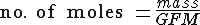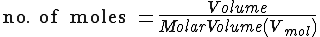2002 - Written Paper - Q12 Question 12

David3001

Posted 30 May 2005 - 03:02 PM

When sodium hydrogencarbonate is heated to 112 degress celsius it decomposes and the gas, carbon dioxide, is given off:

2NaHCO3 (s) ---> Na2CO3 (s) + CO2 (g) + H20 (G)

b) i. Calculate the theoretical volume of carbon dioxide produced by the complete decomposition of 1.68g of sodium hydrogencarbonate.

(Take the molar volume of carbon dioxide to be 23 litres/mol)

---------------------

Any help with this question would be greatly appreciated, thanks.

Ally

Posted 30 May 2005 - 03:12 PM

QUOTE(David3001 @ May 30 2005, 04:02 PM)
When sodium hydrogencarbonate is heated to 112 degress celsius it decomposes and the gas, carbon dioxide, is given off:

2NaHCO3 (s) ---> Na2CO3 (s) + CO2 (g) + H20 (G)

b) i. Calculate the theoretical volume of carbon dioxide produced by the complete decomposition of 1.68g of sodium hydrogencarbonate.

(Take the molar volume of carbon dioxide to be 23 litres/mol)First of all calculate the no. of moles of sodium hydrogencarbonate:= 1.68/84
= 0.02 moles

Since there is a 2 : 1 ratio between 2NaHCO3 and CO2, the no. of moles of CO2 is:

0.01 moles

Now we can find the Volume, by using this relationship:Volume = Molar Volume * no. of moles
= 23 * 0.01
= 230cm3

Volume = 230cm3

TheMesiter

Posted 30 May 2005 - 03:15 PM

my god that was the easiest exam ever
100%

Ally

Posted 30 May 2005 - 03:16 PM

QUOTE(TheMesiter @ May 30 2005, 04:15 PM)

The exams tomorrow.David3001

Posted 30 May 2005 - 03:44 PM

Thanks